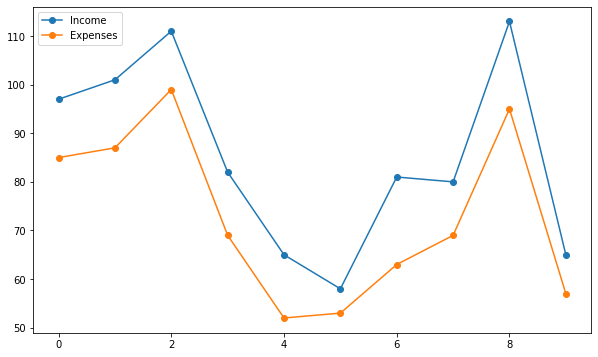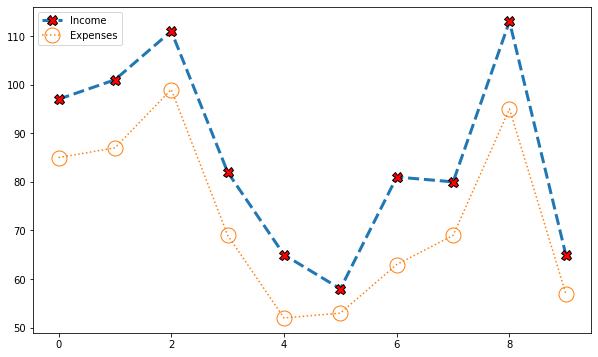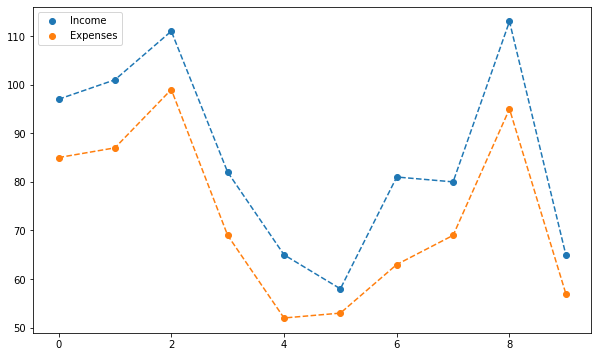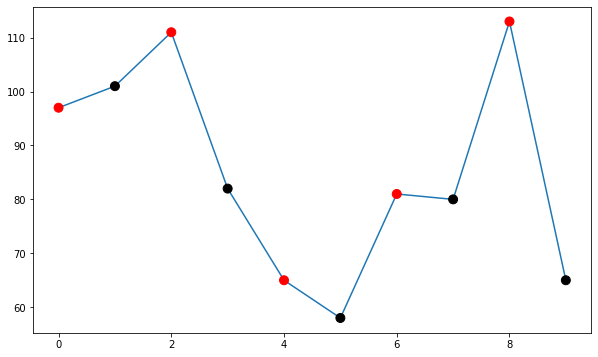## Introduction

Connected scatterplots are just a mix between scatterplots and linecharts. It can be made using the `plot()` function of matplotlib with the possible parameters:

• `x`: The horizontal coordinates of the data points.
• `y`: The vertical coordinates of the data points.
• `linestyle`: Line style, also abbreviated as `ls`. A list of available styles and how to customize them can be found here. Some of the most popular are `"-"` for a solid line, `"--"` for a dashed line, and `":"` for a dotted line.
• `marker`: Marker style. A complete list of available markers can be found here. Some of the most popular are `"o"` for a circle, `"."` for a point, `"^"` for a triangle, etc.

If you want to customize them, just check the scatter and line sections of the website!

## Libraries & Data

``````# Libraries
import matplotlib.pyplot as plt
import numpy as np
import pandas as pd

# Set figure default figure size
plt.rcParams["figure.figsize"] = (10, 6)
# Create a random number generator for reproducibility
rng = np.random.default_rng(1111)

# Get some random points!
x = np.array(range(10))
y = rng.integers(10, 100, 10)
z = y + rng.integers(5, 20, 10)``````

By default, `plt.plot()` generates a solid line plot. This function can also be used to obtain both a scatterplot and a lineplot at once by passing both the `linestyle` and the `marker` type.

## Default grouped connected scatterplot

``````plt.plot(x, z, linestyle="-", marker="o", label="Income")
plt.plot(x, y, linestyle="-", marker="o", label="Expenses")
plt.legend()
plt.show()``````Notice the legend generated automatically combines both lines and markers. This legend also reflects any customization we may apply.

## Customize lines and markers

``````plt.plot(
x, z, ls="--", lw=3,
marker="X", markersize=10, markerfacecolor="red", markeredgecolor="black",
label="Income"
)
plt.plot(
x, y, ls=":",
marker="o", markersize=15, markerfacecolor="None",
label="Expenses"
)
plt.legend()
plt.show()``````## Legend tweaks

If you want to have only either the line or the dot in the legend you can combine `plt.scatter()` and `plt.plot()` giving the label to the one you want to include in the legend. For example:

``````plt.scatter(x, z, label="Income")
plt.plot(x, z, ls="--")

plt.scatter(x, y, label="Expenses")
plt.plot(x, y, ls="--")

plt.legend()
plt.show()``````Constructing the connected scatterplot component by component also gives us more flexibility to customize our plot. For example, it is possible to use different colors for the markers by passing a list of colors to the `color` argument in `plt.scatter()`.

## More customization

``````plt.plot(x, z)
plt.scatter(x, z, color=["red", "black"] * 5, s=80, zorder=10)
plt.show()``````We would have obtained an error if we had passed `color=["red", "black"] * 5` to the `plt.plot()` function.

## Contact & Edit

👋 This document is a work by Yan Holtz. You can contribute on github, send me a feedback on twitter or subscribe to the newsletter to know when new examples are published! 🔥